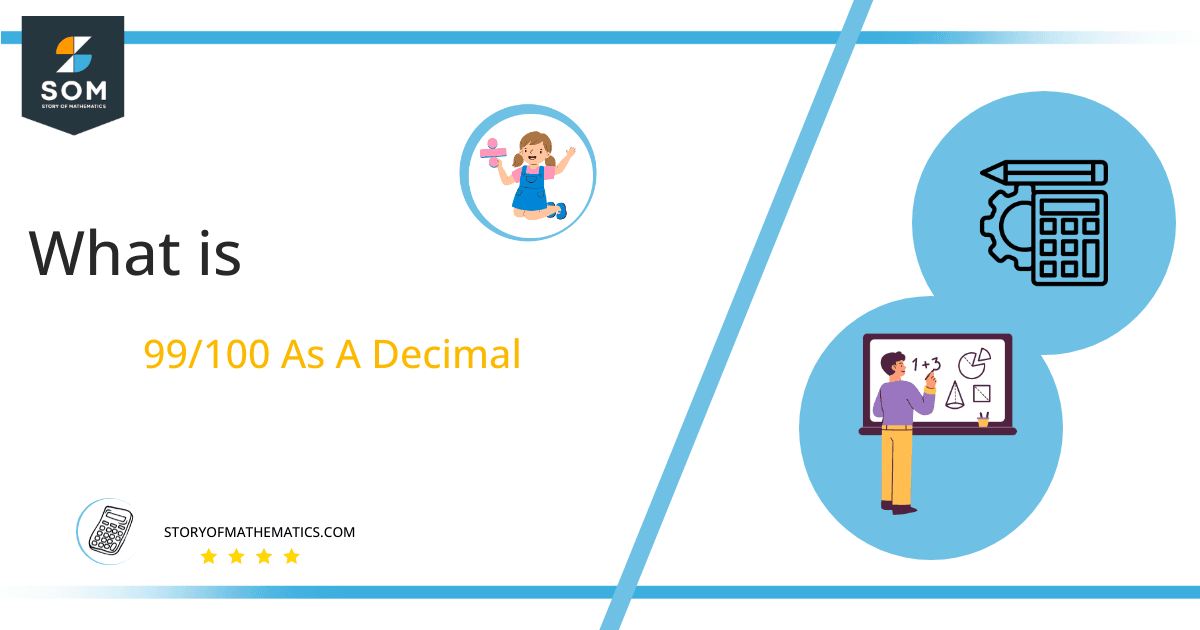# What Is 99/100 as a Decimal + Solution With Free Steps

The fraction 99/100 as a decimal is equal to 0.99.

The fraction 99/100  can be represented as a decimal using the long division method. The fraction is an improper fraction as the denominator is greater than the numerator.

Here, we are more interested in the division types that result in a Decimal value, as this can be expressed as a Fraction. We see fractions as a way of showing two numbers having the operation of Division between them that result in a value that lies between two Integers.Now, we introduce the method used to solve said fraction to decimal conversion, called Long Division, which we will discuss in detail moving forward. So, let’s go through the Solution of fraction 99/100.

## Solution

First, we convert the fraction components, i.e., the numerator and the denominator, and transform them into the division constituents, i.e., the Dividend and the Divisor, respectively.

This can be seen done as follows:

Dividend = 99

Divisor = 100

Now, we introduce the most important quantity in our division process: the Quotient. The value represents the Solution to our division and can be expressed as having the following relationship with the Division constituents:

Quotient = Dividend $\div$ Divisor = 99 $\div$ 100

This is when we go through the Long Division solution to our problem. The Lond Division procedure can be shown in Figure 1 below:Figure 1

## 99/100 Long Division Method

We start solving a problem using the Long Division Method by first taking apart the division’s components and comparing them. As we have 99 and 100, we can see how 99 is Smaller than 100, and to solve this division, we require that 99 be Bigger than 100.

This is done by multiplying the dividend by 10 and checking whether it is bigger than the divisor or not. If so, we calculate the Multiple of the divisor closest to the dividend and subtract it from the Dividend. This produces the Remainder, which we then use as the dividend later.

Now, we begin solving for our dividend 99, which after getting multiplied by 10 becomes 990.

We take this 990 and divide it by 100; this can be seen done as follows:

990 $\div$ 100 $\approx$ 9

Where:

100 x 9 = 900

This will lead to the generation of a Remainder equal to 990 – 900 = 90. Now this means we have to repeat the process by Converting the 90 into 900 and solving for that:

900 $\div$ 100 $=$ 9

Where:

100 x 9 = 900

This, therefore, produces another remainder which is equal to 900 – 900 = 0.

Finally, we have a Quotient generated after combining the three pieces of it as 0.99 = z, with a Remainder equal to 0.Images/mathematical drawings are created with GeoGebra.